# Let's learn math in a fun way ! We will have fun doing a check of the Pythagorean Theorem!

Hello friends of @steemiteducation it's a pleasure to greet you, and on several occasions we have done experiments and activities related to the theme in a fun way so that children get excited and pay attention to what we are going to do in a way that they learn and at the same time enjoy what we do.

Today we will carry out an activity in which we will use a Dominoes game in such a way that our children will love it and at the same time we will check the Pythagorean Theorem.

So before starting the fun we will study what is the Pythagorean Theorem so that the children know what we are talking about..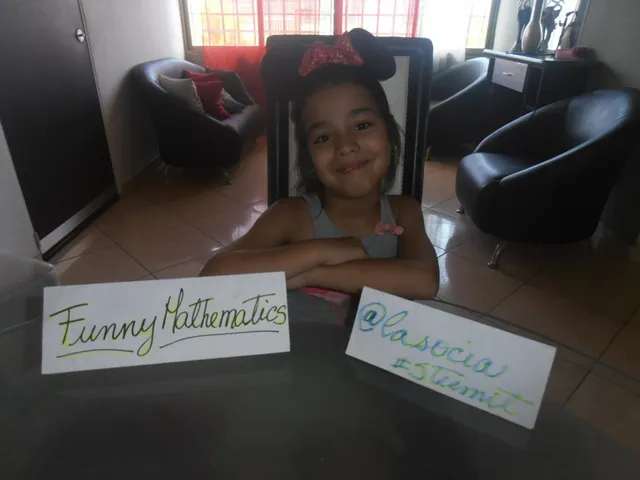# Pythagoras theorem:

Pythagorean Theorem: in every right triangle the square of the hypotenuse (the longest side) is equal to the sum of the squares of the legs (the other two sides of the triangle).

The Pythagorean Theorem can be expressed in another way: the area of ​​the square built on the hypotenuse of a right triangle is equal to the sum of the areas of the squares built on the legs. Source

# Materials:

• A game of Dominoes.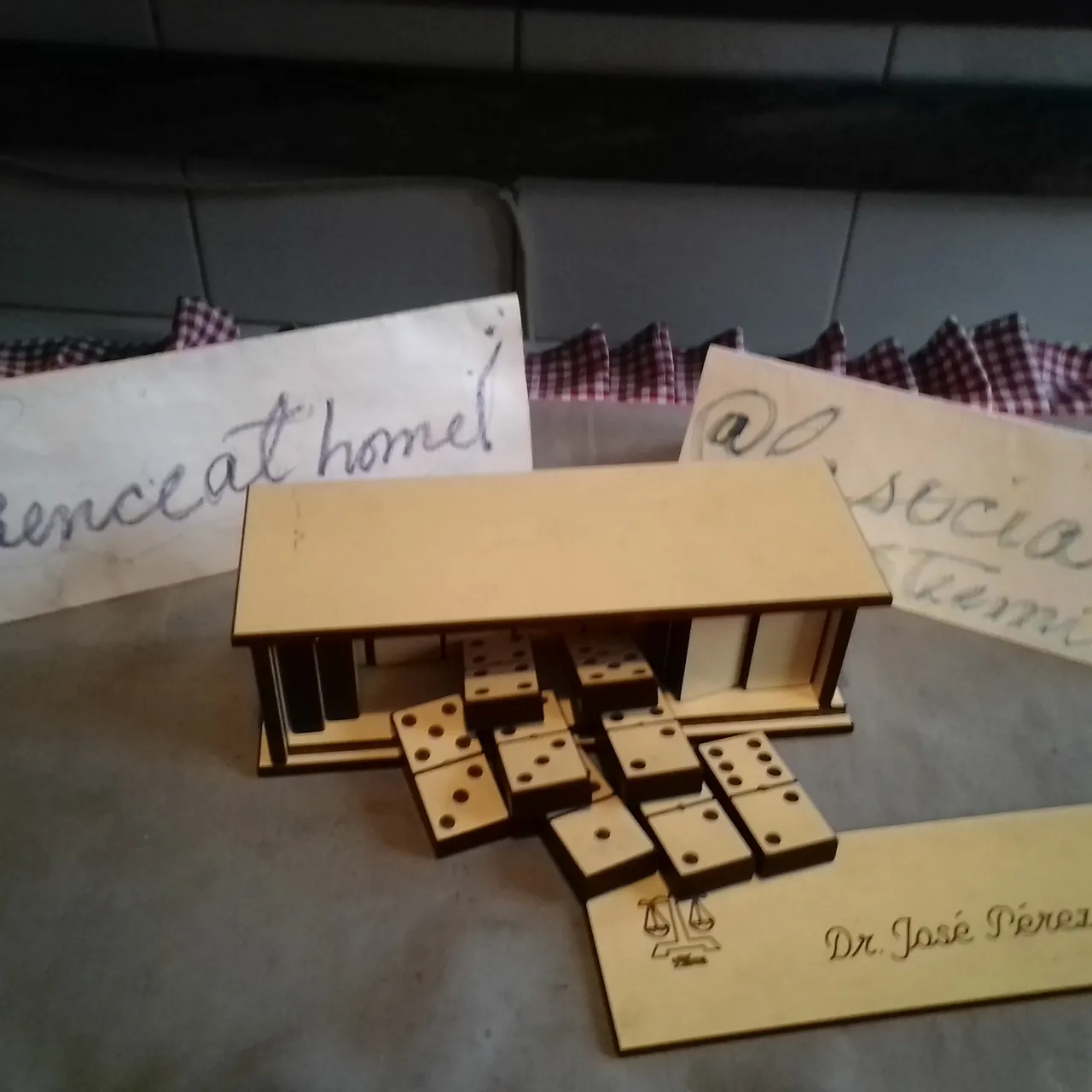# Process

• With a few dominoes and a right triangle you can make a very curious verification of the Pythagorean Theorem.

• The sides of the right triangle have 3, 4 and 5 units. One half of a domino will be taken as a unit. Then the dominoes are placed on the squares.

• The square built on the leg of 3 units contains 9 squares.

• The square built on the leg of 4 units contains 16 squares.

• And the square built on the hypotenuse has 25 squares that is equal to the sum of 9 +16.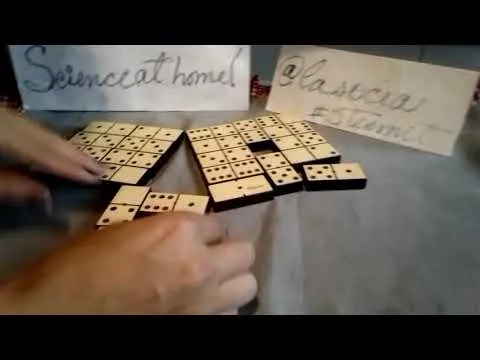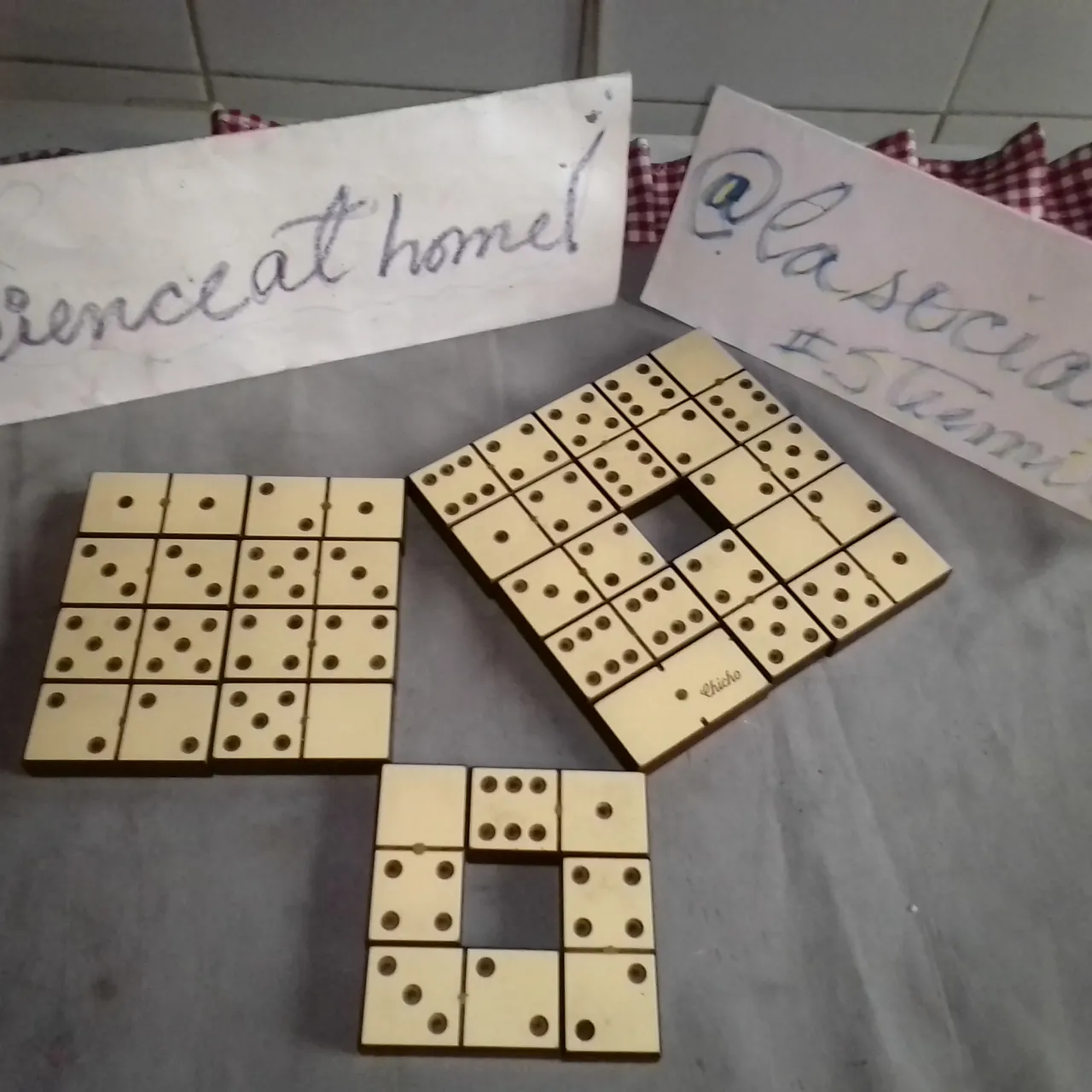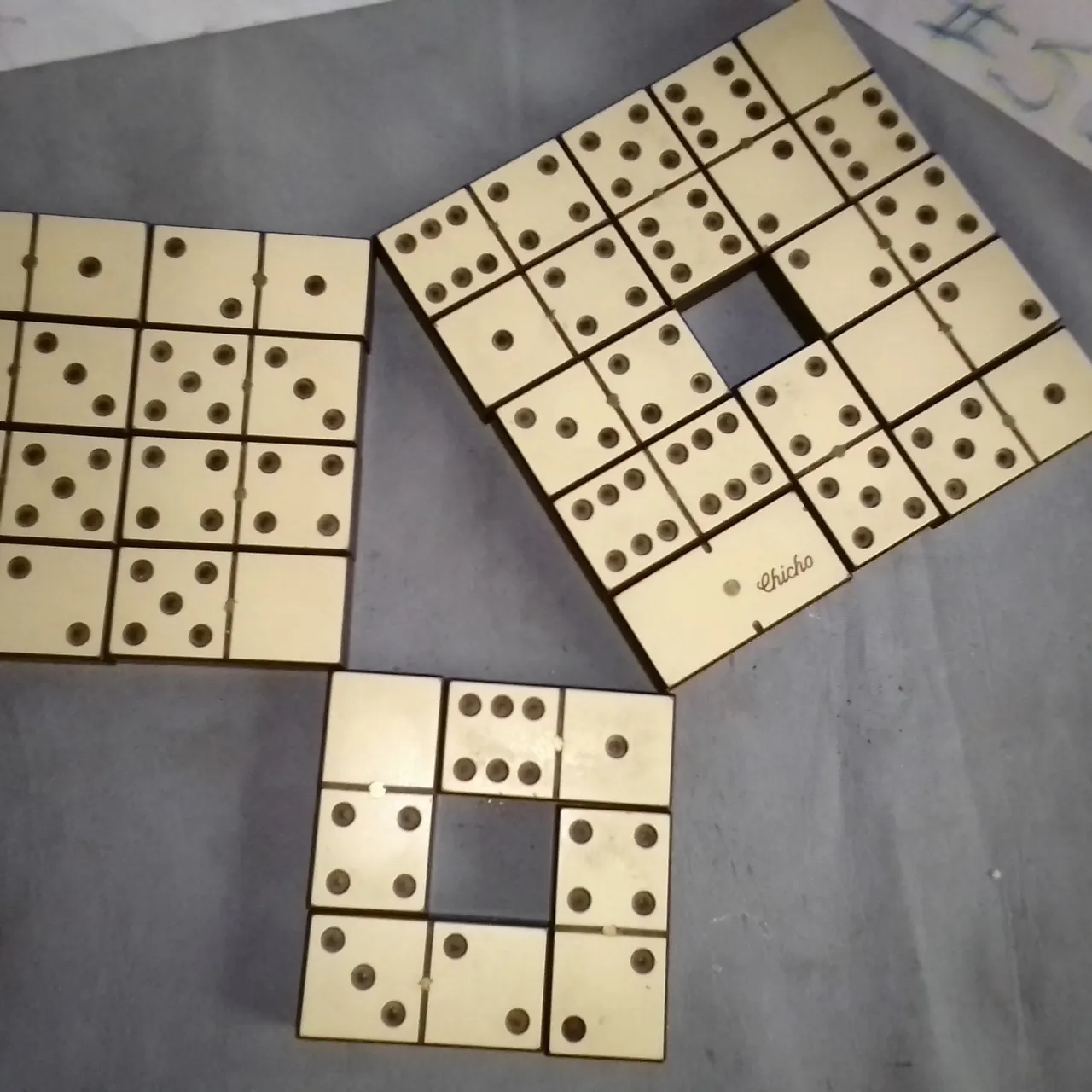Most children consider mathematics to be boring and complicated, mathematics is not just numbers and equations, we, as parents, have to look for the best teaching and learning strategies we can and it is the idea of ​​these publications that you know and the Once you motivate yourself to find a way to educate your children in a fun way and, at the same time, share a quality time with them, I invite you to put it into practice.

Thank you for visiting my blog and I hope to see you in my next publication, where we will surely educate our children in a fun and dynamic way. Regards!!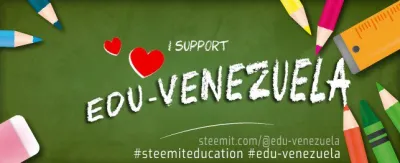H2
H3
H4
3 columns
2 columns
1 column
1 Comment